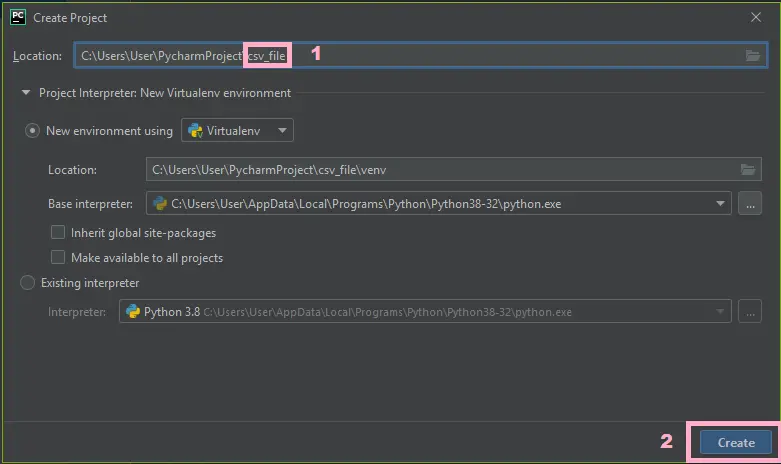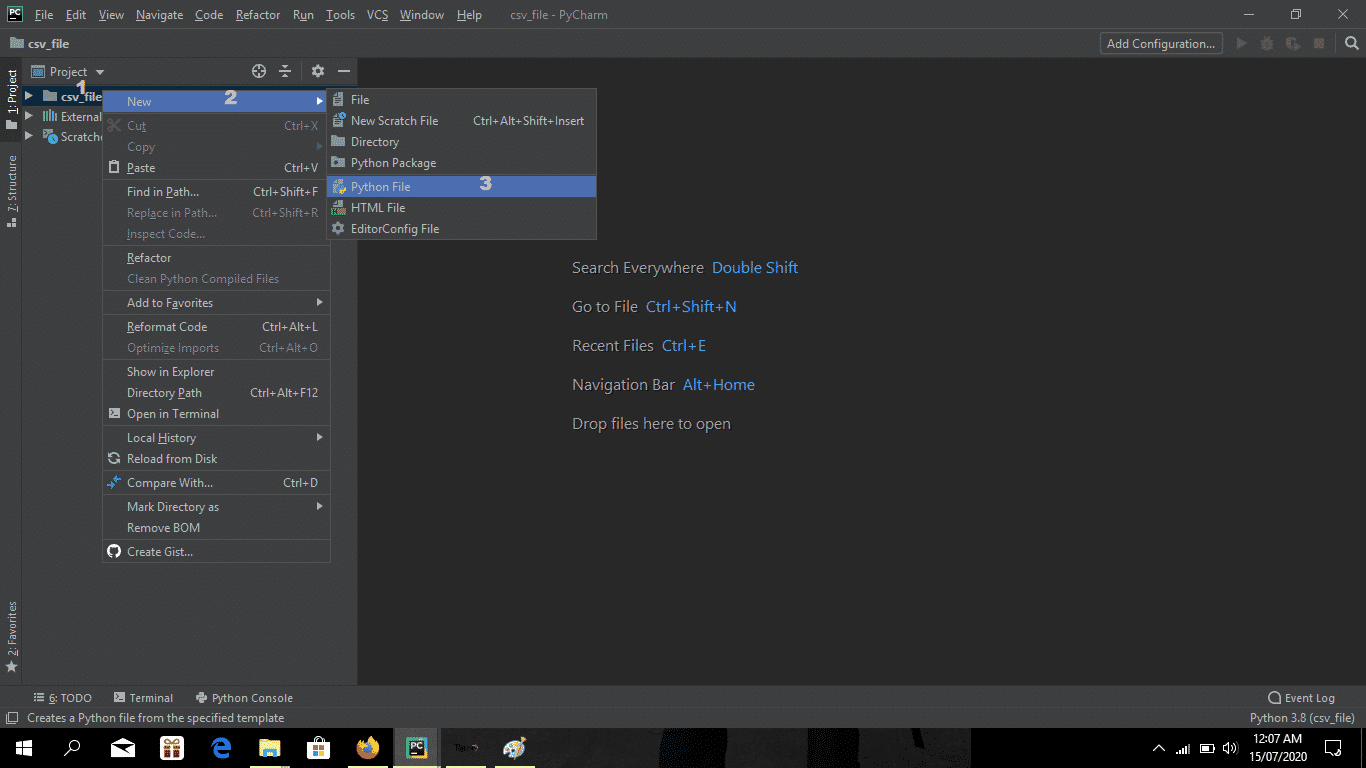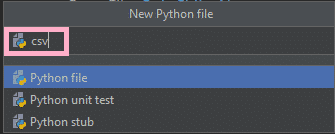How To Read Multiple Columns From CSV File In Python - 2022

# How To Read Multiple Columns From CSV File In Python

This article about How To Read Multiple Columns From CSV File In Python is design in python programming language. Python is very smooth to research the syntax emphasizes readability and it is able to reduces time ingesting in developing.

## About The Project on How To Read Multiple CSV Files in Python

In this tutorial, I will teach you on How To Read A CSV File In Python, Also in this tutorial is the simplest way for the beginners or the student to Writing CSV File In Python .

To start ceating a CSV File In Python, make sure that you have Pycharm IDE installed in your computer.

## Recommendations

By the way if you are new to python programming and you don’t know what would be the the Python IDE to use, I have here a list of Best Python IDE for Windows, Linux, Mac OS that will suit for you.

## Steps on How To Read Multiple Columns From CSV File In Python

Time needed: 5 minutes.

How To Read CSV File In Python With Source Code

• Step 1: Create a project name.

First, open Pycharm IDE and click “file” after that create a project name and then click “create” button.• Step 2: Create python file.

Second “right click” your project name folder and choose new and then click “python file“.• Step 3: Name your python file.

Third, name your python file and click “enter” to start creating CSV File In Python.• Step 4: The actual code.

Fourth, the actual coding in creating CSV File In Python, you are free to copy the code and download full source code given below.

## The code given below is for Importing modules.

``````from tkinter import *
import tkinter.ttk as ttk
import csv``````

In this part of codes which is importing all the modules given on top.

## The code given below is for the design of this project.

``````root = Tk()
root.title("Python - Import CSV File To Tkinter Table")
width = 500
height = 400
screen_width = root.winfo_screenwidth()
screen_height = root.winfo_screenheight()
x = (screen_width/2) - (width/2)
y = (screen_height/2) - (height/2)
root.geometry("%dx%d+%d+%d" % (width, height, x, y))
root.resizable(0, 0)

TableMargin = Frame(root, width=500)
TableMargin.pack(side=TOP)
scrollbarx = Scrollbar(TableMargin, orient=HORIZONTAL)
scrollbary = Scrollbar(TableMargin, orient=VERTICAL)
tree = ttk.Treeview(TableMargin, columns=("Firstname", "Lastname", "Address"), height=400, selectmode="extended", yscrollcommand=scrollbary.set, xscrollcommand=scrollbarx.set)
scrollbary.config(command=tree.yview)
scrollbary.pack(side=RIGHT, fill=Y)
scrollbarx.config(command=tree.xview)
scrollbarx.pack(side=BOTTOM, fill=X)
tree.column('#0', stretch=NO, minwidth=0, width=0)
tree.column('#1', stretch=NO, minwidth=0, width=200)
tree.column('#2', stretch=NO, minwidth=0, width=200)
tree.column('#3', stretch=NO, minwidth=0, width=300)
tree.pack()``````

In this module which is the design of the project CSV File In Python.

## The code given below is for reading the CSV file.

``````with open('test.csv') as f:
firstname = row['firstname']
lastname = row['lastname']

In this module which is reading the CSV file and displayed in tkinter table.

## CompleteSource Code

``````from tkinter import *
import tkinter.ttk as ttk
import csv

root = Tk()
root.title("Python - Import CSV File To Tkinter Table")
width = 500
height = 400
screen_width = root.winfo_screenwidth()
screen_height = root.winfo_screenheight()
x = (screen_width/2) - (width/2)
y = (screen_height/2) - (height/2)
root.geometry("%dx%d+%d+%d" % (width, height, x, y))
root.resizable(0, 0)

TableMargin = Frame(root, width=500)
TableMargin.pack(side=TOP)
scrollbarx = Scrollbar(TableMargin, orient=HORIZONTAL)
scrollbary = Scrollbar(TableMargin, orient=VERTICAL)
tree = ttk.Treeview(TableMargin, columns=("Firstname", "Lastname", "Address"), height=400, selectmode="extended", yscrollcommand=scrollbary.set, xscrollcommand=scrollbarx.set)
scrollbary.config(command=tree.yview)
scrollbary.pack(side=RIGHT, fill=Y)
scrollbarx.config(command=tree.xview)
scrollbarx.pack(side=BOTTOM, fill=X)
tree.column('#0', stretch=NO, minwidth=0, width=0)
tree.column('#1', stretch=NO, minwidth=0, width=200)
tree.column('#2', stretch=NO, minwidth=0, width=200)
tree.column('#3', stretch=NO, minwidth=0, width=300)
tree.pack()

with open('test.csv') as f:
firstname = row['firstname']
lastname = row['lastname']

#============================INITIALIZATION==============================
if __name__ == '__main__':
root.mainloop()``````